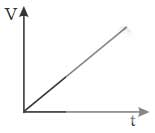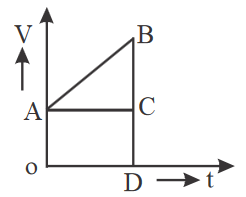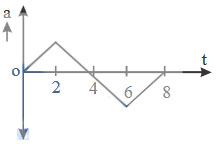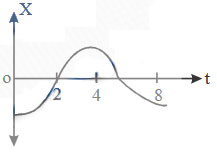51.

Here are the graphs of $$v\rightarrow t$$ of a moving body. Which of them is not suitable ?

(1).(2).(3).(4).A. 1

B. 2

C. 3

D. 4

52.In the figure there is a graph of velocity $$\rightarrow$$ time for a particle. Which area shows the displacement covered by the particle after time t ?

A. Closed fig. AODCA

B. Closed fig. ABCA

C. Closed fig. AODCBA

D. none of above

53.In the figure there is a graph of velocity $$\rightarrow$$ time for a particle. Which part shows initial velocity of the particle ?

A. OA

B. AB

C. AC

D. AOA

54.In the figure there is a graph of velocity $$\rightarrow$$ time for a particle. How will you calculate the acceleration of the particle ?

A. taking length of AB

B. taking magnitude of BC

C. taking slope of AC

D. taking slope of AB

55.Given graph shows relation between position and time. Find correct graph of acceleration $$\rightarrow$$ time.1.2.3.4

A. 1

B. 2

C. 3

D. 4